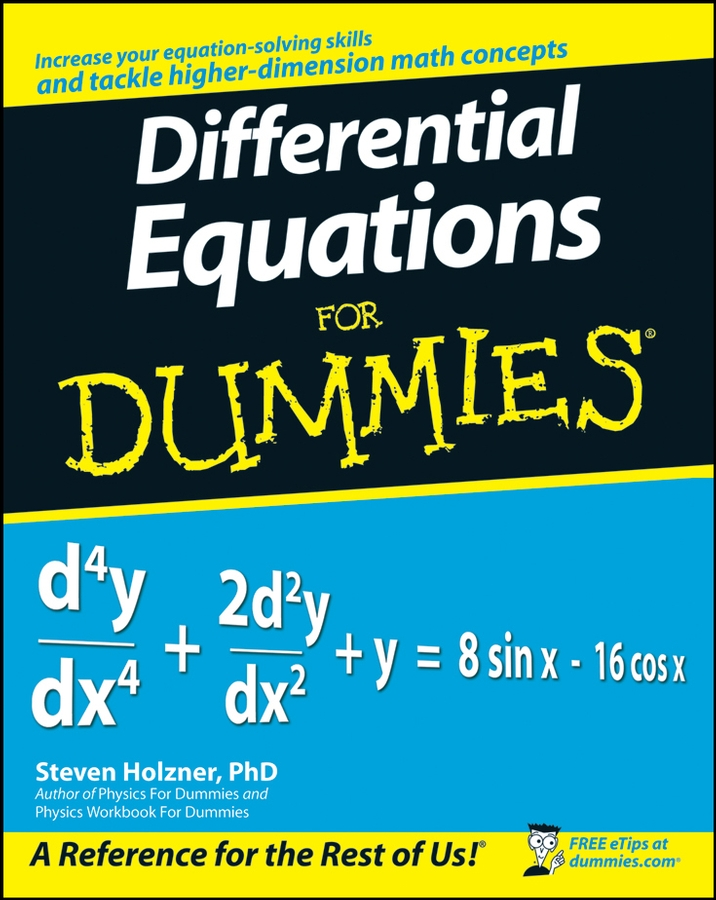##### Differential Equations For DummiesThe English system of measurement is most commonly used in the United States (but, ironically, not in England). Although you’re probably familiar with most of the English units of measurement, in the following list, you can see the most important ones. The list also shows some equivalent values that can help you do conversions from one type of unit to another.
• Units of distance: Distance — also called length — is measured in inches (in.), feet (ft.), yards (yd.), and miles (mi.):

12 inches = 1 foot

3 feet = 1 yard

5,280 feet = 1 mile

• Units of fluid volume: Fluid volume (also called capacity) is the amount of space occupied by a liquid such as water, milk, or wine. Volume is measured in fluid ounces (fl. oz.), cups (c.), pints (pt.), quarts (qt.), and gallons (gal.):

8 fluid ounces = 1 cup

2 cups = 1 pint

2 pints = 1 quart

4 quarts = 1 gallon

Units of fluid volume are typically used for measuring the volume of things that can be poured. The volume of solid objects is more commonly measured in cubic units of distance such as cubic inches, cubic feet, and so forth.

• Units of weight: Weight is the measurement of how strongly gravity pulls an object toward Earth. Weight is measured in ounces (oz.), pounds (lb.), and tons.

16 ounces = 1 pound

2,000 pounds = 1 ton

Don’t confuse fluid ounces, which measure volume, with ounces, which measure weight. These units are two completely different types of measurements!

• Units of time: Time is hard to define, but everybody knows what it is. Time is measured in seconds, minutes, hours, days, weeks, and years:

60 seconds = 1 minute

60 minutes = 1 hour

24 hours = 1 day

7 days = 1 week

365 days ≈ 1 year

The conversion from days to years is approximate, because earth’s daily rotation on its axis and its yearly revolution around the sun aren’t exactly synchronized. A year is closer to 365.25 days, which is why leap years exist.

Months are left out of the picture because the definition of a month is imprecise — it can vary from 28 to 31 days.

• Unit of speed: Speed is the measurement of how much time an object takes to move a given distance. The most common unit of speed is miles per hour (mph).

• Unit of temperature: Temperature measures how much heat an object contains. This object can be a glass of water, a turkey in the oven, or the air surrounding your house. Temperature is measured in degrees Fahrenheit (°F).